Class 10 RD Sharma Solutions – Chapter 5 Trigonometric Ratios – Exercise 5.1 | Set 2

• Last Updated : 21 Feb, 2021

(ii) cot2θ

Solution:

cotθ = 7/8 = Base/Perpendicular

In right-angled ΔPQR,

∠Q = 90°, PQ = 8, RQ = 7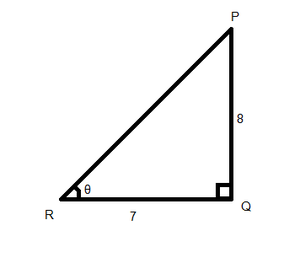Using Pythagoras Theorem

PR2 = PQ2 + QR2

PR2 = 82 + 72 = 64 + 49

PR2 = 113

PR = √113
Now

sinθ = Perpendicular/Hypotenuse = PQ/PR = 8/√113

cosθ = Base/Hypotenuse = QR/PR = 7/√113

(i)Putting the values of sinθ and cosθ in the equation=== 49/64

(ii) cot2θ

= (cosθ/sinθ)2

Putting the values of sinθ and cosθ in the following equation

=== 49/64

or

cot2θ = (cotθ)2 = (7/8)2 = 49/64

Question 8. If 3 cot A = 4, check whetheror not.

Solution:

Given, 3cot A = 4 or cot A = 4/3

Draw a △ ABC where ∠B = 90°, AB = 4, BC = 3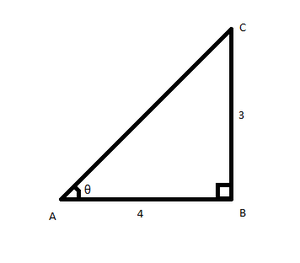Using Pythagoras Theorem

AC2 = AB2 + BC2

AC2 = 42 + 32 = 16 + 9

AC2 = 25

AC = 5

Now,

Taking LHS

===== 7/25

Taking RHS

= cos2A – sin2A

=(=(=(= 7/25

RHS = LHS (Hence Proved)

Question 9. If tanθ = a/b, find the value ofSolution:

Given, tanθ = a/b

Draw a △ABC where ∠B = 90°, AB = b, BC = a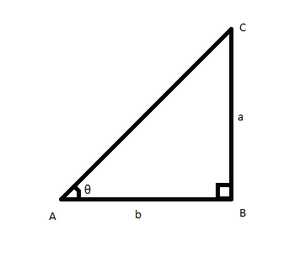Using Pythagoras Theorem

AC2 = BC2 + AB2

AC2 = a2 + b2

AC2Now,=== (b + a)/(b – a)

Question 10. If 3tanθ = 4, find the value ofSolution:

Given tanθ = 4/3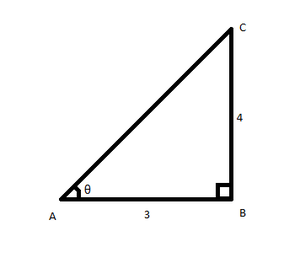Now, Dividing the numerator and denominator by cosθ

=Putting the values of tanθ in the above equation

=== 8/10

= 4/5

Question 11. If 3cotθ = 2, find the value ofSolution:

Given: 3cotθ = 2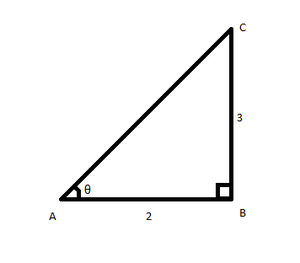Using Pythagoras Theorem

AC2 = BC2 + AB2

AC2 = 32 + 42

AC2 = 9 + 16 = 25

AC = 5

Now,

== 6/18 = 1/3

Question 12. If tanθ = a/b, prove thatSolution:

Given, tanθ = a/b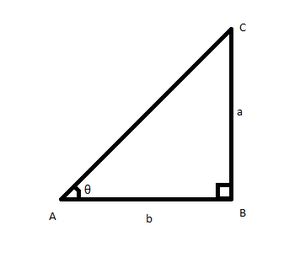Using Pythagoras Theorem

AC2 = BC2 + AB2

AC2 = a2 + b2

AC2Now,

=Putting the values of sinθ and cosθ in the above equation

====Hence Proved

Question 13. If secθ = 13/5, prove that=3

Solution:

Given, secθ = 13/5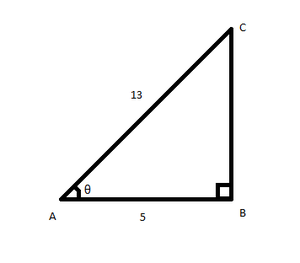Using Pythagoras Theorem

AC2 = BC2 + AB2

132 = BC2 + 52

BC2 = 169 – 25 = 144

BC = 12

Now,

Taking LHS

=Putting the values of sinθ and cosθ in the above equation= 3 = RHS

Hence Proved

Question 14. If cosθ = 12/13, show that sinθ(1 – tanθ) = 35/156

Solution:

We have cosθ = 12/13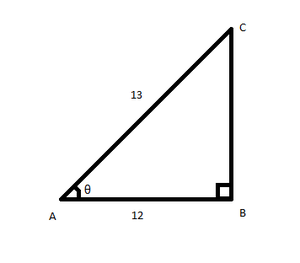Using Pythagoras Theorem

AC2 = BC2 + AB2

132 = BC2 + 122

BC2 = 169 – 144 = 25

BC = 5

Now,

Taking LHS

= sinθ(1 – tanθ)

=== 35/156

= RHS

Hence Proved

Question 15. If cotθ = 1/√3, show thatSolution:

Given, cotθ = 1/√3

tanθ = 1/cotθ =√3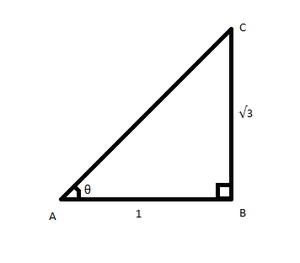From Pythagoras theorem,

AC2 = AB2 + BC2

AC2 = 12 + (√3)2

AC2 = 3 + 1 = 4

AC = 2

Now,

Taking LHS

=== 3/5

Hence Proved

Question 16. If tanθ = 1/√7, thenSolution:

We have

tanθ = 1/√7

cotθ = √7

We know sec2θ = (1 + tan2θ) = 1 + 1/7 = 8/7

and cosec2θ = (1 + cot2θ) = 1 + 7 = 8

Now,== 48/64 = 3/4

Question 17. If secθ = 5/4, find the value ofSolution:

Given:

secθ = 5/4

cosθ = 1/secθ = 4/5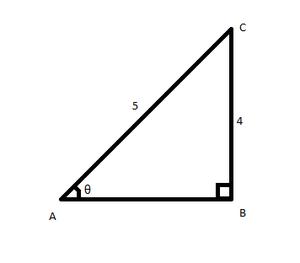From Pythagoras theorem,

AC2 = BC2 + AB2

52 = BC2 + 42

BC2 = 25 − 16 = 9

BC = 3

Now,

=== 12/7

Question 18. If tanθ = 12/13, find the value ofSolution:

Given: tanθ = 12/13

From Pythagoras theorem,

AC2 = BC2 + AB2

AC2 = (13)2 + (12)2

AC2 = 313

AC = √313

sinθ = 12/√313

cosθ = 13/√313

We have= \= 312/25

Question 19. If cosθ = 3/5, then evaluateSolution:

Given:

cosθ = 3/5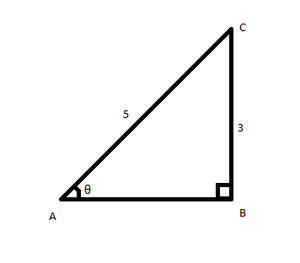From Pythagoras theorem,

AC2 = BC2 + AB2

52 = 32 + AB2

AB2 = 25 − 9 = 16

AB = 4

Now==== (1/20) × (3/8)

= 3/160

Question 20. If sinθ = 3/5, then evaluateSolution:

Given,

sinθ = 3/5

Now====== (sinθ – 1)/(2)

Putting the value of sinθ, we get

=== -1/5

Question 21. If tanθ = 24/7, find that sinθ + cosθ.

Solution:

Given: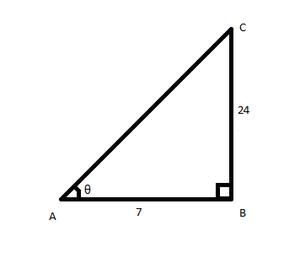From Pythagoras theorem,

AC2 = BC2 + AB2

AC2 = 242 + 72

AC2 = 576 + 49 = 625

AC = 25

Now,

= sinθ + cosθ

= 24/25 + 7/25

= (24 + 7)/25

= 31/25

My Personal Notes arrow_drop_up